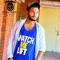Units Unit Name Marks I NUMBER SYSTEMS 6 II ALGEBRA 20 III COORDINATE GEOMETRY 6 IV GEOMETRY 15 V TRIGONOMETRY 12 VI MENSURATION 10 VII STATISTICS & PROBABILTY 11 Total 80

## UNIT I: NUMBER SYSTEMS

1. REAL NUMBERS
Euclid’s division lemma, Fundamental Theorem of Arithmetic - statements after reviewing work done earlier and after illustrating and motivating through examples, Proofs of irrationality of 2, 3, 5 Decimal representation of rational numbers in terms of terminating/non-terminating recurring decimals.

## UNIT II: ALGEBRA

1. POLYNOMIALS
Zeros of a polynomial. Relationship between zeros and coefficients of quadratic polynomials. Statement and simple problems on division algorithm for polynomials with real coefficients.

2. PAIR OF LINEAR EQUATIONS IN TWO VARIABLES
Pair of linear equations in two variables and graphical method of their solution, consistency/inconsistency. Algebraic conditions for number of solutions. Solution of a pair of linear equations in two variables algebraically - by substitution, by elimination and by cross multiplication method. Simple situational problems. Simple problems on equations reducible to linear equations.

Standard form of a quadratic equation ax 2 + bx + c = 0, (a ≠ 0). Solutions of quadratic equations (only real roots) by factorization, by completing the square and by using quadratic formula. Relationship between discriminant and nature of roots.
Situational problems based on quadratic equations related to day to day activities to be incorporated.
4. ARITHMETIC PROGRESSIONS
Motivation for studying Arithmetic Progression Derivation of the nth term and sum of the first n terms of A.P. and their application in solving daily life problems.

## UNIT III: COORDINATE GEOMETRY

1. LINES (In two-dimensions)
Review: Concepts of coordinate geometry, graphs of linear equations. Distance formula. Section formula (internal division). Area of a triangle.

## UNIT IV: GEOMETRY

1.TRIANGLES
Definitions, examples, counter examples of similar triangles.

1. (Prove) If a line is drawn parallel to one side of a triangle to intersect the other two sides in distinct points, the other two sides are divided in the same ratio.
2. (Motivate) If a line divides two sides of a triangle in the same ratio, the line is parallel to the third side.
3. (Motivate) If in two triangles, the corresponding angles are equal, their corresponding sides are proportional and the triangles are similar.
4. (Motivate) If the corresponding sides of two triangles are proportional, their corresponding angles are equal and the two triangles are similar.
5. (Motivate) If one angle of a triangle is equal to one angle of another triangle and the sides including these angles are proportional, the two triangles are similar.
6. (Motivate) If a perpendicular is drawn from the vertex of the right angle of a right triangle to the hypotenuse, the triangles on each side of the perpendicular are similar to the whole triangle and to each other.
7. (Prove) The ratio of the areas of two similar triangles is equal to the ratio of the squares of their corresponding sides.
8. (Prove) In a right triangle, the square on the hypotenuse is equal to the sum of the squares on the other two sides.
9. (Prove) In a triangle, if the square on one side is equal to sum of the squares on the other two sides, the angles opposite to the first side is a right angle.

2. CIRCLES
Tangent to a circle at, point of contact

1. (Prove) The tangent at any point of a circle is perpendicular to the radius through the point of contact.
2. (Prove) The lengths of tangents drawn from an external point to a circle are equal

3. CONSTRUCTIONS

1. Division of a line segment in a given ratio (internally).
2. Tangents to a circle from a point outside it.
3. Construction of a triangle similar to a given triangle.

## UNIT V: TRIGONOMETRY

1. INTRODUCTION TO TRIGONOMETRY
Trigonometric ratios of an acute angle of a right-angled triangle. Proof of their existence (well defined); motivate the ratios whichever are defined at 0O and 90O. Values (with proofs) of the trigonometric ratios of 30O, 45O and 60O. Relationships between the ratios.

2. TRIGONOMETRIC IDENTITIES
Proof and applications of the identity sin2A + cos2A = 1. Only simple identities to be given. Trigonometric ratios of complementary angles.

3. HEIGHTS AND DISTANCES : Angle of elevation, Angle of Depression.
Simple problems on heights and distances. Problems should not involve more than two right triangles. Angles of elevation / depression should be only 30°, 45° 60°.

UNIT VI: MENSURATION

1. AREAS RELATED TO CIRCLES
Motivate the area of a circle; area of sectors and segments of a circle. Problems based on areas and perimeter / circumference of the above said plane figures. (In calculating area of segment of a circle, problems should be restricted to central angle of 60°, 90° and 120° only. Plane figures involving triangles, simple quadrilaterals and circle should be taken.)

2. SURFACE AREAS AND VOLUMES
1. Surface areas and volumes of combinations of any two of the following: cubes, cuboids, spheres, hemispheres and right circular cylinders/cones. Frustum of a
cone.
2. Problems involving converting one type of metallic solid into another and other mixed problems. (Problems with combination of not more than two different solids
be taken)

## UNIT VII: STATISTICS AND PROBABILITY

1. STATISTICS
Mean, median and mode of grouped data (bimodal situation to be avoided). Cumulative frequency graph.

2. PROBABILITY
Classical definition of probability. Simple problems on single events (not using set notation)